# RS Aggarwal Solutions for Class 6 Maths Chapter 18 Circles

RS Aggarwal Solutions for Class 6 Chapter 18 Circles are provided here in PDF format. Students who practice RS Aggarwal Solutions for Class 6 Chapter 18 will boost their confidence in Mathematics and helps them to solve problems with ease. Practicing RS Aggarwal textbook problems help students improve their knowledge and score high marks.

BYJU’S experts have solved RS Aggarwal textbook questions for Class 6 Chapter 18 in simple PDF format. Download PDF of Class 6 Chapter 18 in their respective links.

## Download PDF of RS Aggarwal Solutions for Class 6 Chapter 18 – Circles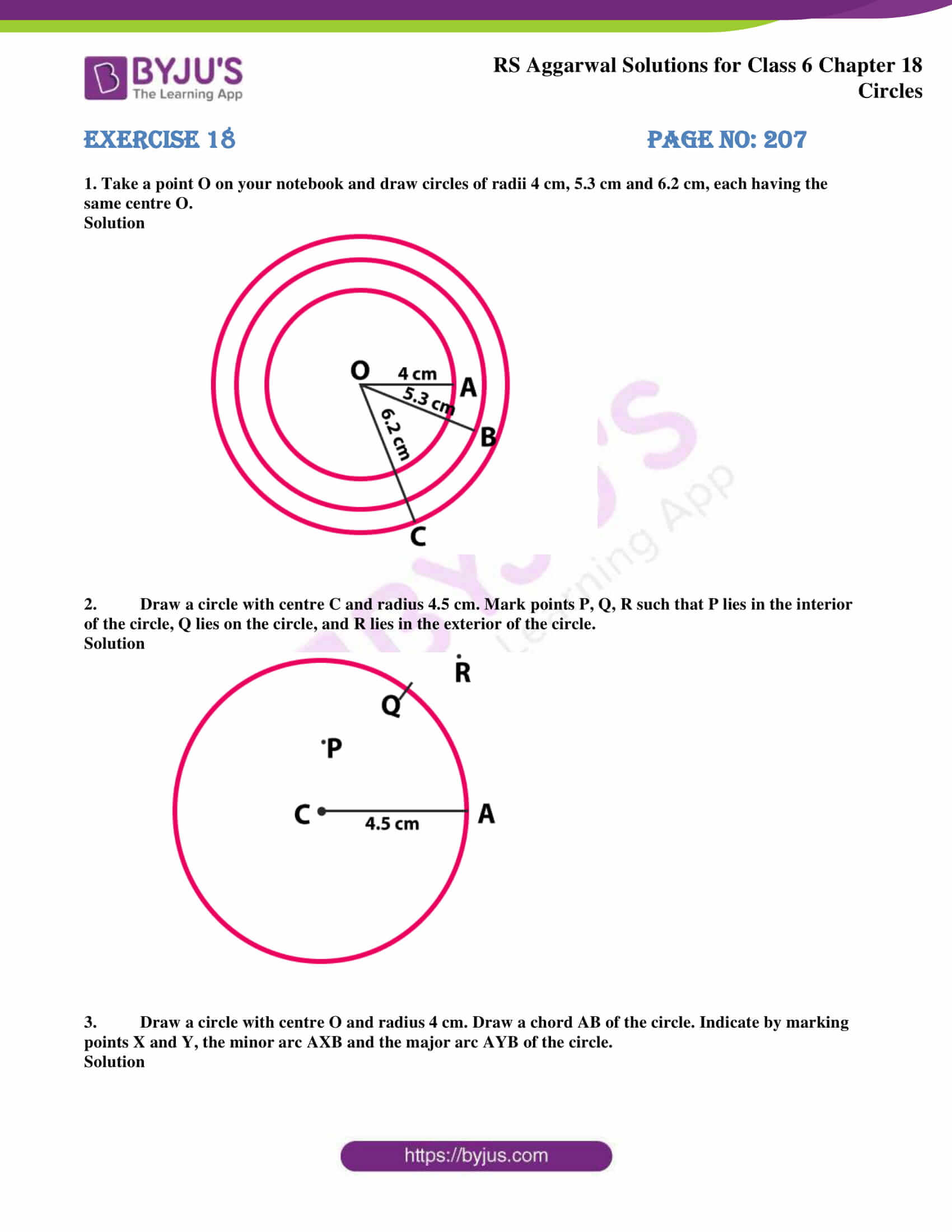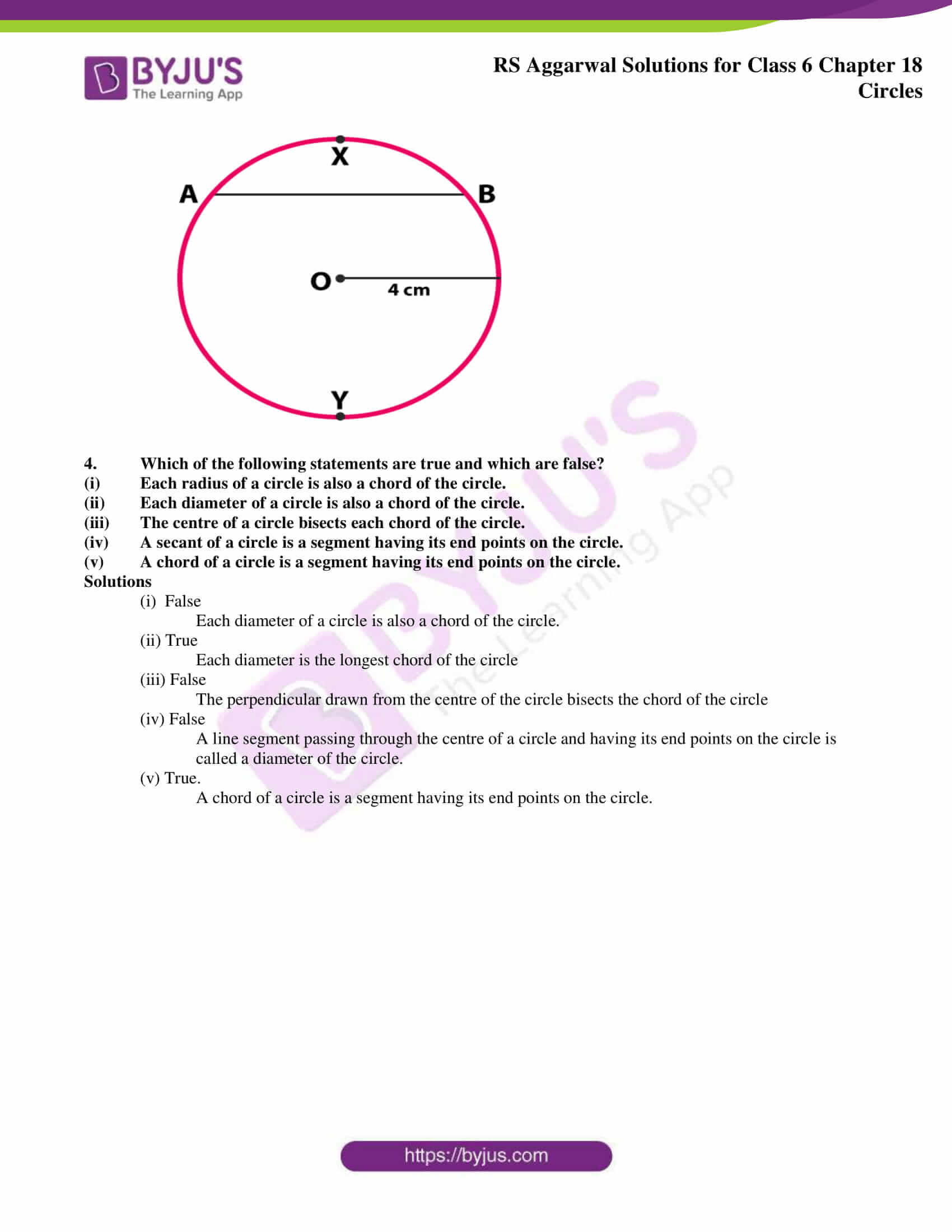### Access answers to chapter 18 – Circles

Exercise 18 PAGE NO: 207

1. Take a point O on your notebook and draw circles of radii 4 cm, 5.3 cm and 6.2 cm, each having the same centre O.

Solution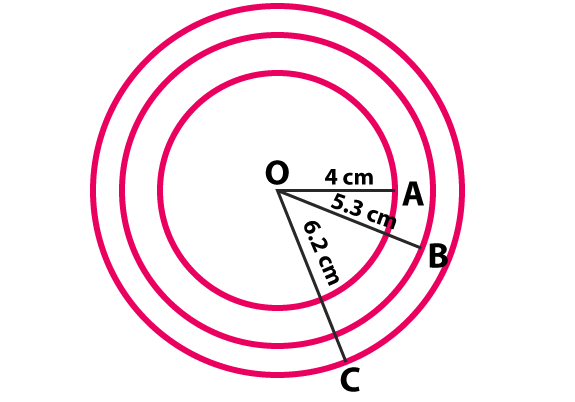2. Draw a circle with centre C and radius 4.5 cm. Mark points P, Q, R such that P lies in the interior of the circle, Q lies on the circle, and R lies in the exterior of the circle.

Solution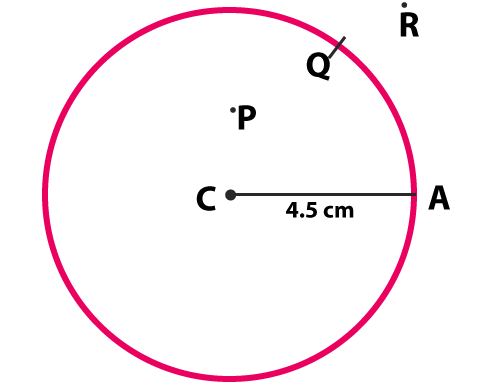3. Draw a circle with centre O and radius 4 cm. Draw a chord AB of the circle. Indicate by marking points X and Y, the minor arc AXB and the major arc AYB of the circle.

Solution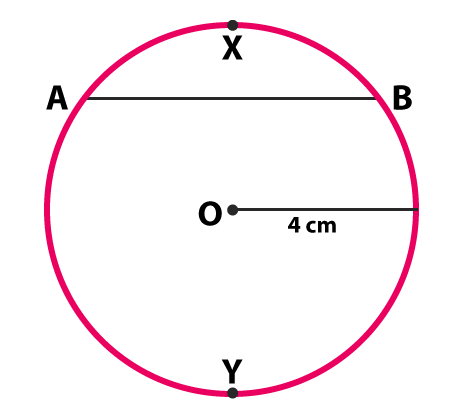4. Which of the following statements are true and which are false?

(i) Each radius of a circle is also a chord of the circle.

(ii) Each diameter of a circle is also a chord of the circle.

(iii) The centre of a circle bisects each chord of the circle.

(iv) A secant of a circle is a segment having its end points on the circle.

(v) A chord of a circle is a segment having its end points on the circle.

Solutions

(i) False

Each diameter of a circle is also a chord of the circle.

(ii) True

Each diameter is the longest chord of the circle

(iii) False

The perpendicular drawn from the centre of the circle bisects the chord of the circle

(iv) False

A line segment passing through the centre of a circle and having its end points on the circle is called a diameter of the circle.

(v) True.

A chord of a circle is a segment having its end points on the circle.

### RS Aggarwal Solutions for Class 6 Chapter 18 Circles

Chapter 18 includes 1 exercise. Questions from RS Aggarwal textbook about Circles has been solved step by step. Let’s check out the topics which are talked about in this chapter

• Circle
• Interior and exterior of a Circle
• Diameter of a Circle
• Chord of a Circle
• Secant of a Circle
• Circumference of a Circle
• Segments of a Circle
• Semicircle
• Arc
• Sector of a Circle
• Concentric Circles

Also, access RS Aggarwal Solutions for Class 6 Chapter 18 Exercise

### Chapter Brief of RS Aggarwal Solutions for Class 6 Maths Chapter 18 – Circles

Circle is a simple closed shape where all points in a plane at a given distance from the centre. The distance around a Circle is called the circumference. The distance between the midpoint and the circle border is called the radius. A line segment that joins two points present on a curve is the chord. A line which is drawn across a circle passing through the centre is called diameter. Bicycle wheel, coin, bangles are the examples of Circle.